# Efficiency of different stimulus ensembles for systems identification¶

In reverse correlation, we approximate a nonlinear, stochastic function $y \sim f(X)$ locally by a linear approximation weighted by a gaussian window. We compute this approximation by computing the response $y$ of the system for a variety of normally distributed inputs $X \sim N(0, \sigma^2)$. An estimate of the response is given by a sum of the stimuli weighted by the responses, $\hat w = \frac{1}{N} X^T y$. When these responses are action potentials, or spikes measures from biological neurons, the estimate $\hat w$ is also called the spike-triggered average.

How good is this estimator? Not very good. It can, however, be significantly improved by careful consideration of the properties of this Monte Carlo estimator, namely by changing the input ensemble to use antithetic sampling, or by shifting the distribution of the response.

To demonstrate this, I use an example nonlinear function to be estimated via this black-box method. It consists of weighting the input with a windowed sinusoid, followed by an expansive nonlinearity that drives a Poisson process.

In :
%config InlineBackend.figure_format = 'retina'
import numpy as np
import matplotlib.pyplot as plt
import pandas as pd
import plotnine
import seaborn as sns

sns.set(style="darkgrid")

class LNP:
"""A simple LNP model neuron."""
def __init__(self):
rg = np.arange(-31.5, 32.5)
self.w = np.cos(rg / 20.0 * 2 * np.pi) * np.exp(-rg ** 2 / 2 / 10 ** 2)
self.w /= 4
self.input_size = len(self.w)
self.rate_multiplier = 1

def forward(self, X):
"""The nonlinearity is $\log(1 + \exp(x))$"""
return np.random.poisson(self.rate_multiplier * np.log(1 + np.exp(X.dot(self.w) - .5)))

In :
N = 10000
model = LNP()

plt.plot(model.w)
plt.title("Model weights")

Out:
Text(0.5, 1.0, 'Model weights')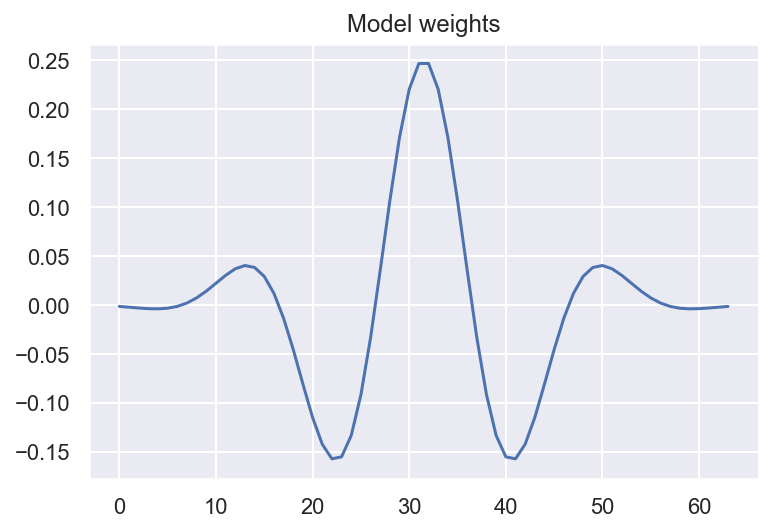In :
X = np.random.randn(N, model.input_size)
Y = model.forward(X)

# Measure the convergence of the model as a function of N
plt.plot(Y)

Out:
[<matplotlib.lines.Line2D at 0x7f8d036a9910>]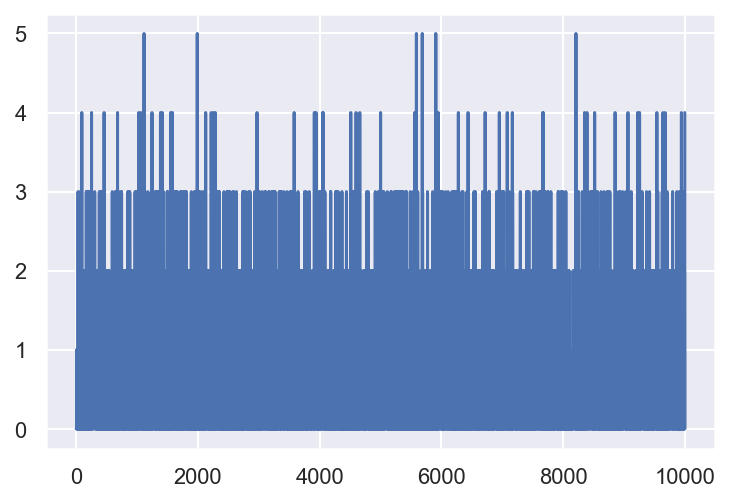Calculate estimates of the weights using reverse correlation. This is given by:

$$\frac{1}{N} X^T y$$
In :
def estimate_w_hat(X, Y):
N = X.shape
# remove the mean.
estimates = np.cumsum(X * (Y.reshape((-1, 1))), axis=0)
w_hat = 1 / np.arange(1, N + 1).reshape((-1, 1)) * estimates
return w_hat

def calculate_rho(w_hats, w):
rhos = w_hats.dot(w) / np.sqrt((w_hats ** 2).sum(1) * (w ** 2).sum())
assert rhos.shape == w_hats.shape[:1]
return rhos

In :
w_hat = estimate_w_hat(X, Y)
rho = calculate_rho(w_hat, model.w)

plt.plot(np.arange(1, N+1), rho)
plt.xlabel("Number of stimuli")
plt.ylim((0, 1))
plt.title("correlation between estimated weights and true weights")

/home/pmin/miniconda3/envs/brian-notebooks/lib/python3.7/site-packages/ipykernel_launcher.py:9: RuntimeWarning: invalid value encountered in true_divide
if __name__ == '__main__':

Out:
Text(0.5, 1.0, 'correlation between estimated weights and true weights')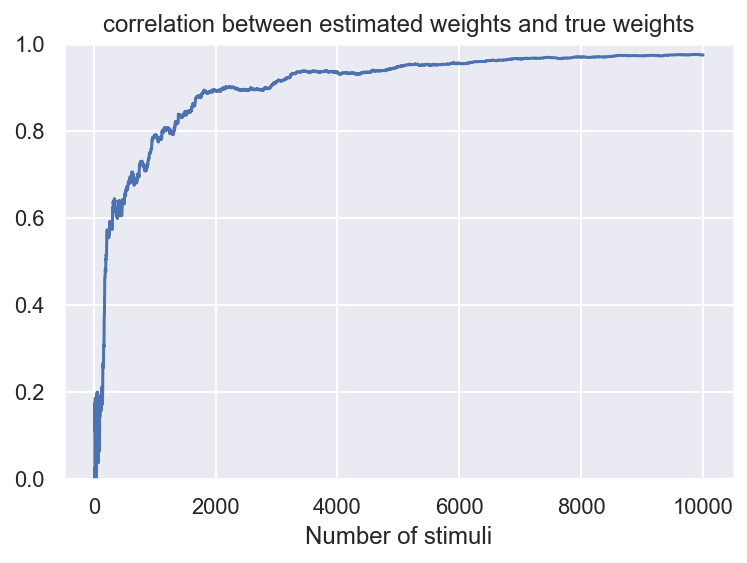## Improving on the reverse correlation estimate¶

Our crude estimate works, but we can squeeze a bit more efficiency out of it.

One concern is that our estimate is unduly affected by shifts in the mean of the response. If we set:

$y \to y + a$

Then the reverse correlation estimate shifts to:

$$\hat w = \frac{1}{N} X^T(y + a) = \frac{1}{N} X^T y + \frac{a}{N} X^T \hat 1$$

Now imagine that our responses $y$ are centered around 0. As $a$ becomes larger and larger, the variance of $\hat w$ will grow, and our estimates will be worse and worse. Let's show this in a simulation:

In :
results = []
X = np.random.randn(N, len(model.w))
y = model.forward(X)
# Center y for this demo.
y = y - y.mean()
for i in range(5):
w_hat = estimate_w_hat(X, y + i * 2)
rho = calculate_rho(w_hat, model.w)
results += [{'nstims': j + 1,
'rho': rho[j],
'offset': str(i*2)} for j in range(len(rho))]

In :
df = pd.DataFrame(results)
sns.lineplot(x='nstims', y='rho', hue='offset', data=df, ci=None)
plt.legend((0, 2, 4, 6, 8))

Out:
<matplotlib.legend.Legend at 0x7f8d026068d0>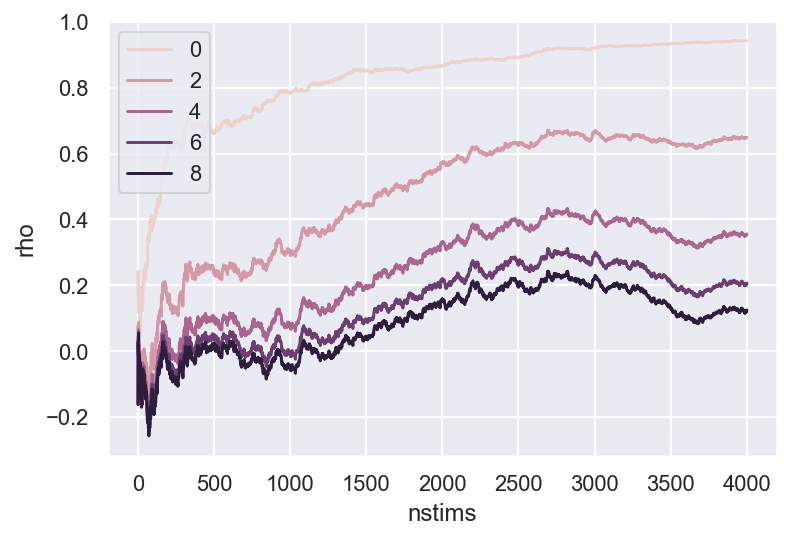Adding an offset increases the variance of the estimates and thus it takes more stimuli for the reverse correlation estimate to converge to the true underlying parameter. One solution is antithetic sampling. The idea is to generate stimuli in symmetric pairs $(x, -x)$. It follows that we have that $X^T \hat 1 = 0$, which reduces the variance of the estimate:

In :
results = []
X = np.random.randn(N, len(model.w))

rg = np.floor(np.arange(N) / 2.0).astype(np.int)
alternating_sign = 2 * ((np.arange(N) % 2) - .5)
Xs = alternating_sign.reshape((-1, 1)) * X[rg, :]

y = model.forward(Xs)

# Center y for this demo.
y = y - y.mean()
for i in range(5):
w_hat = estimate_w_hat(Xs, y + i * 2)
rho = calculate_rho(w_hat, model.w)
results += [{'nstims': j + 1,
'rho': rho[j],
'offset': str(i*2)} for j in range(len(rho))]

df = pd.DataFrame(results)
sns.lineplot(x='nstims', y='rho', hue='offset', data=df, ci=None)
plt.legend((0, 2, 4, 6, 8))
plt.title("Estimate quality, antithetic sampling estimate")

Out:
Text(0.5, 1.0, 'Estimate quality, antithetic sampling estimate')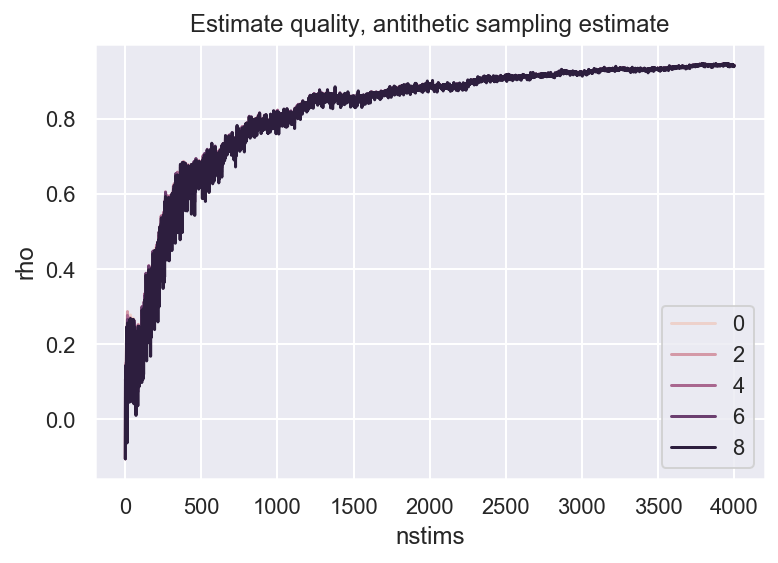Indeed, this renders the estimates largely immune to the offset. However, there's another way of reducing the variance: choose $a$ such that $Var(\hat w)$ is minimized. Note that the expectation of $X^T a$ is 0, so it cannot affect the mean of the estimator; however, it can nevertheless change its variance. Indeed, we can show that the minimum variance estimate for $\hat w$ occurs when $a = \bar y$.

This trick also pops up in other Monte Carlo estimates; see the Control Variates section of this excellent blog post for more references.

In :
def estimate_w_hat_low_var(X, Y):
N = X.shape
# remove the mean.
Ym = np.cumsum(Y)
estimates = np.cumsum(X * (Y.reshape((-1, 1))), axis=0)
mean = (Ym / np.arange(1, N + 1)).reshape((-1, 1)) * np.cumsum(X, axis=0)
w_hat = 1 / np.arange(1, N + 1).reshape((-1, 1)) * (estimates - mean)
return w_hat

results = []
X = np.random.randn(N, len(model.w))
y = model.forward(X)

for i in range(5):
w_hat = estimate_w_hat_low_var(X, y + i * 2)
rho = calculate_rho(w_hat, model.w)
results += [{'nstims': j + 1,
'rho': rho[j],
'offset': str(i*2)} for j in range(len(rho))]

/home/pmin/miniconda3/envs/brian-notebooks/lib/python3.7/site-packages/ipykernel_launcher.py:9: RuntimeWarning: invalid value encountered in true_divide
if __name__ == '__main__':

In :
df = pd.DataFrame(results)
sns.lineplot(x='nstims', y='rho', hue='offset', data=df, ci=None)
plt.legend((0, 2, 4, 6, 8))
plt.title("Estimate quality, variance-stabilized estimate")

Out:
Text(0.5, 1.0, 'Estimate quality, variance-stabilized estimate')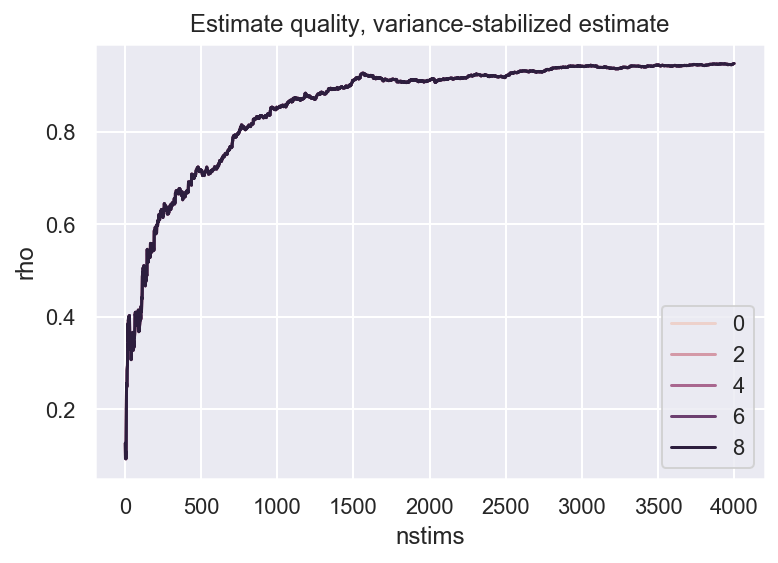Which trick works best? For this scenario, it turns out that the variance-stabilized estimate works better than antithetic sampling:

In :
N = 4000
results = []
for i in range(100):
X = np.random.randn(N, model.input_size)
Y = model.forward(X)

w_hat = estimate_w_hat_low_var(X, Y)
rho = calculate_rho(w_hat, model.w)

rg = np.floor(np.arange(N) / 2.0).astype(np.int)
alternating_sign = 2 * ((np.arange(N) % 2) - .5)
Xs = alternating_sign.reshape((-1, 1)) * X[rg, :]
Y = model.forward(Xs)

w_hat = estimate_w_hat_low_var(Xs, Y)
rho_p = calculate_rho(w_hat, model.w)

results += [{'run': i,
'nstims': j + 1,
'rho': rho[j],
'sampling_type': 'normal'} for j in range(len(rho))]
results += [{'run': i,
'nstims': j + 1,
'rho': rho_p[j],
'sampling_type': 'antithetical'} for j in range(len(rho_p))]

df = pd.DataFrame(results)

/home/pmin/miniconda3/envs/brian-notebooks/lib/python3.7/site-packages/ipykernel_launcher.py:9: RuntimeWarning: invalid value encountered in true_divide
if __name__ == '__main__':

In :
ax = plt.figure(figsize=(8, 6)).gca()
sns.lineplot(x='nstims', y='rho', hue='sampling_type', data=df[::100], ax=ax)

Out:
<matplotlib.axes._subplots.AxesSubplot at 0x7f8d02f32dd0>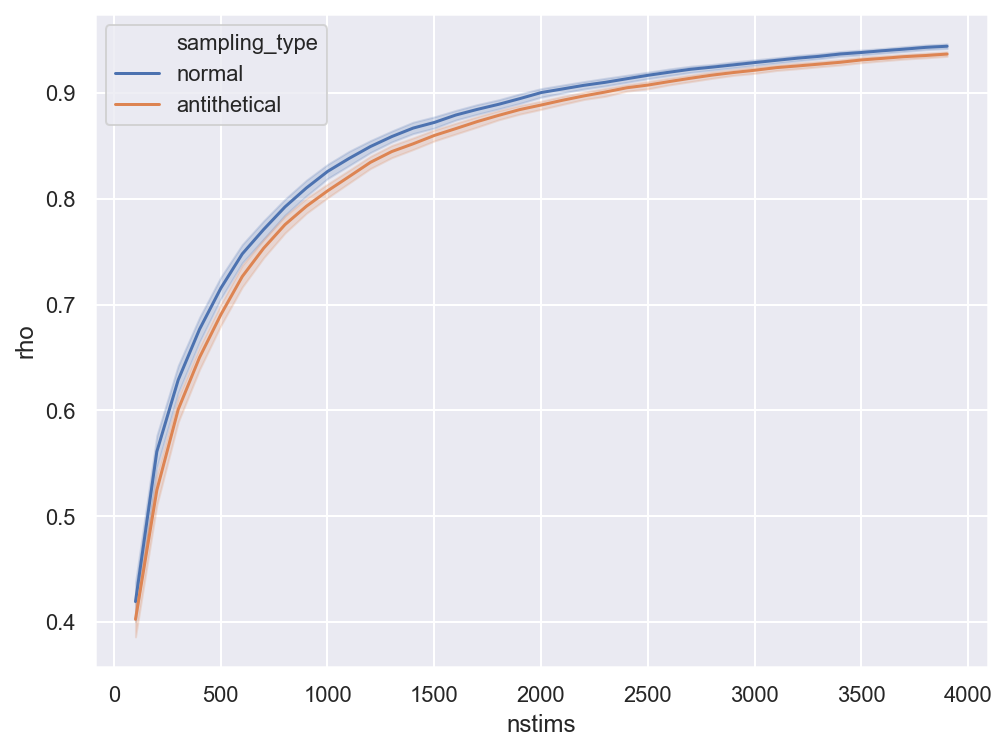Conclusion: always use the variance-stabilized estimate by removing subtracting the mean response from $y$. Antithetic sampling may also be helpful in some scenarios.